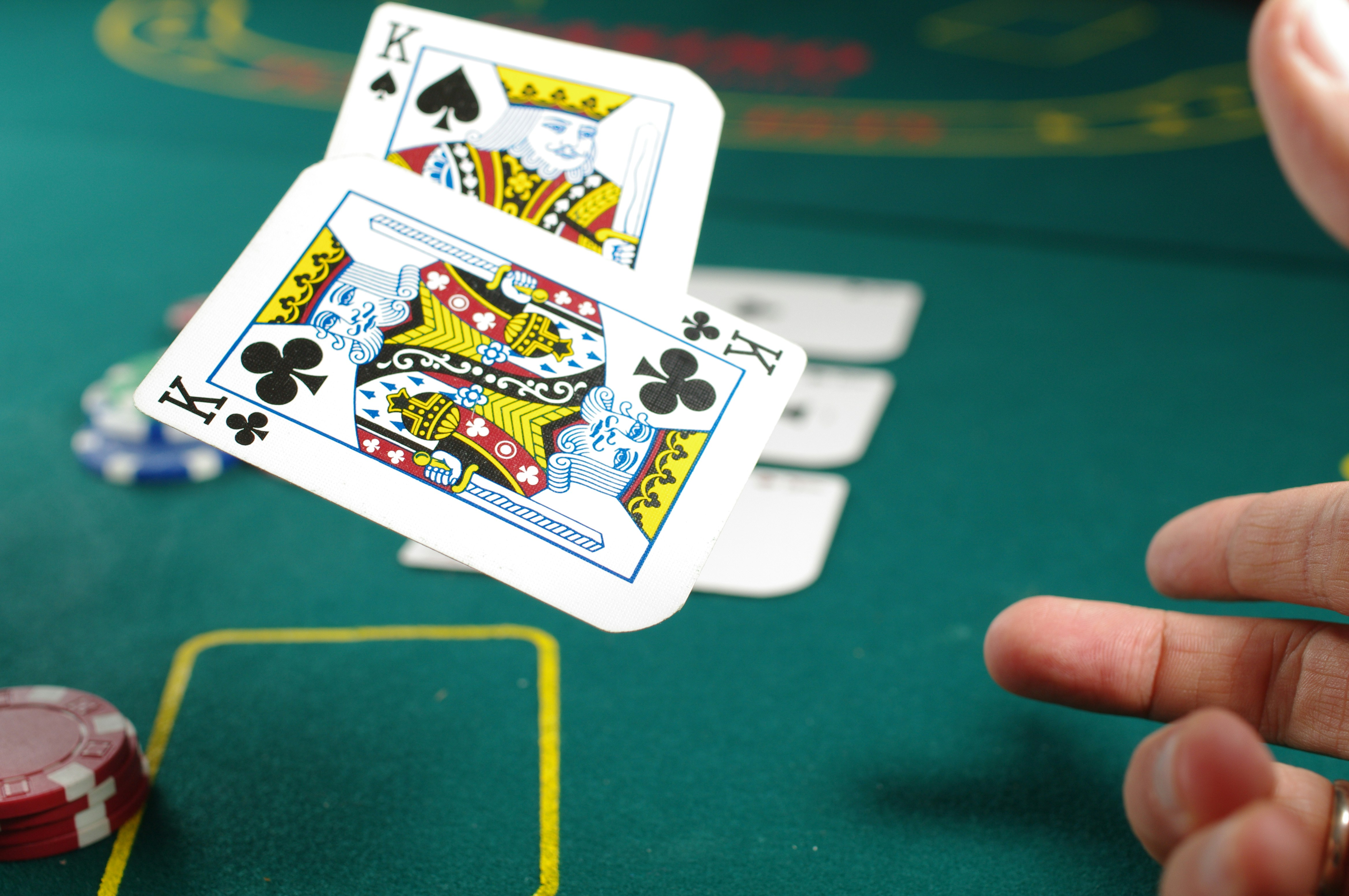## The Fisher-Yates Shuffle

Published on in Algorithms## The question is quite simple

You are given a list, how can you perform an in-place shuffle of the list such that each element in the list is equally likely to end up in either spot in the final list?

A common idea would be to walk through the list and swap each element with another random element. But does that work? Let's find out.

Assume we have a list of 3 elements `[a, b, c]` on which we want to perform the shuffle. With our current approach, we'll have to swap element at each index with another random element.
That means, for all 3 positions we have 3 random choices. That gives us a total of 27 set of choices.

## This does not give us a uniform distribution!

If we have 3 (unique) elements in our list, then the total permutations that we have are 3! (factorial), which is 6. We can list them out as –

`[a, b, c]`
`[a, c, b]`
`[b, a, c]`
`[b, c, a]`
`[c, a, b]`
`[c, b, a]`

But our 27 possible set of choices does not equally distribute into 6 possible permutations! That means, some of our possible outcomes will be more probable than others.

## The Fisher-Yates shuffle (aka the Knuth shuffle)

We simply select one item randomly from the list with equal probability and fix it to be the first item. We skip the first item, and select another random item from the list and fix it as the second item. We repeat the process until the last item (second-last to be precise) is fixed to its position.

We can quite easily prove the uniformity of this shuffle with a little knowledge of probability. Assume that our list has `n` elements.

Probability of an item to be picked first –
Since we have `n` elements, and each element is equally likely. An element to be the first element has the probability `1/n`.

Probability of an item to be picked second –
Now, we are only left with `n-1` elements. The probability of an element being picked second is dependent on the outcome of the first event.
`P(not picked first, picked second) = [(n - 1) / n] * [1 / (n - 1)]`
`P(second) = 1/n`.

We can continue to check for third, fourth and so on. We will find that the probability remains `1/n`. Interesting!

## Let's write some code!

The code runs in `O(n)` time and `O(1)` space.